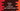# Python program to print a mirrored right-angle star triangle### Introduction:

In this post, we will learn how to print a mirrored right-angle triangle pattern in Python. For this example, I will use star (*) to print the pattern but you can also use any other character to print that.

The final output will look as like below:

``````	    *
*  *
*  *  *
*  *  *  *
*  *  *  *  *``````

It looks complex but actually it is easy. Let me explain to you a bit more:

### How to write the program:

Let’s take a look at the below pattern:

``````#  #  #  #  *
#  #  #  *  *
#  #  *  *  *
#  *  *  *  *
*  *  *  *  *``````

Can we print this ?

• It’s height or size is 5
• For row 1, we are printing 5 - 1 = 4 # and one *
• For row 2, we are printing 5 - 2 = 3 # and two *
• For row 3, we are printing 5 - 3 = 2 # and three *

Easy ?

So, if you print one blank space ’ ’ instead of ’#‘, you will get the above mirrored right angled triangle. Just write two loops and use the above logic 🙂

### Using for loop:

Below is the program that uses for loop to print the mirrored right angled triangle pattern in python:

``````height = int(input("Enter the height of the triangle : "))

for i in range(1, height + 1):
for j in range(1, height + 1):
if(j <= height - i):
print(' ', end = '  ')
else:
print('*', end = '  ')
print()``````
• We are taking the height of the triangle as input from the user and storing it in height variable.
• We have two for loops. The outer loop is for the rows of the triangle and inner loop is for the columns of the triangle.
• i is used for the outer loop and j is for the inner loop. We are checking if j is less than or equal to height - i, we are printing a blank space and else we are printing ***.

### Sample Output:

``````Enter the height of the triangle : 5
*
*  *
*  *  *
*  *  *  *
*  *  *  *  *

Enter the height of the triangle : 10
*
*  *
*  *  *
*  *  *  *
*  *  *  *  *
*  *  *  *  *  *
*  *  *  *  *  *  *
*  *  *  *  *  *  *  *
*  *  *  *  *  *  *  *  *
*  *  *  *  *  *  *  *  *  *``````

Python program to print a Mirrored right angled triangle star pattern

### Writing the same program using while loop:

``````height = int(input("Enter the height of the triangle : "))

i = 1

while(i < height + 1):
j = 1
while(j < height + 1):
if(j <= height - i):
print(' ', end = '  ')
else:
print('*', end = '  ')
j = j + 1
i = i + 1
print()``````

This program is same as the previous one. The only difference is the place we are initializing the variables i and j.

• Before starting the program, we are initializing i as 1.
• In the outer while loop, we are initializing j as 1 before starting the inner while loop.

This program will print similar output.